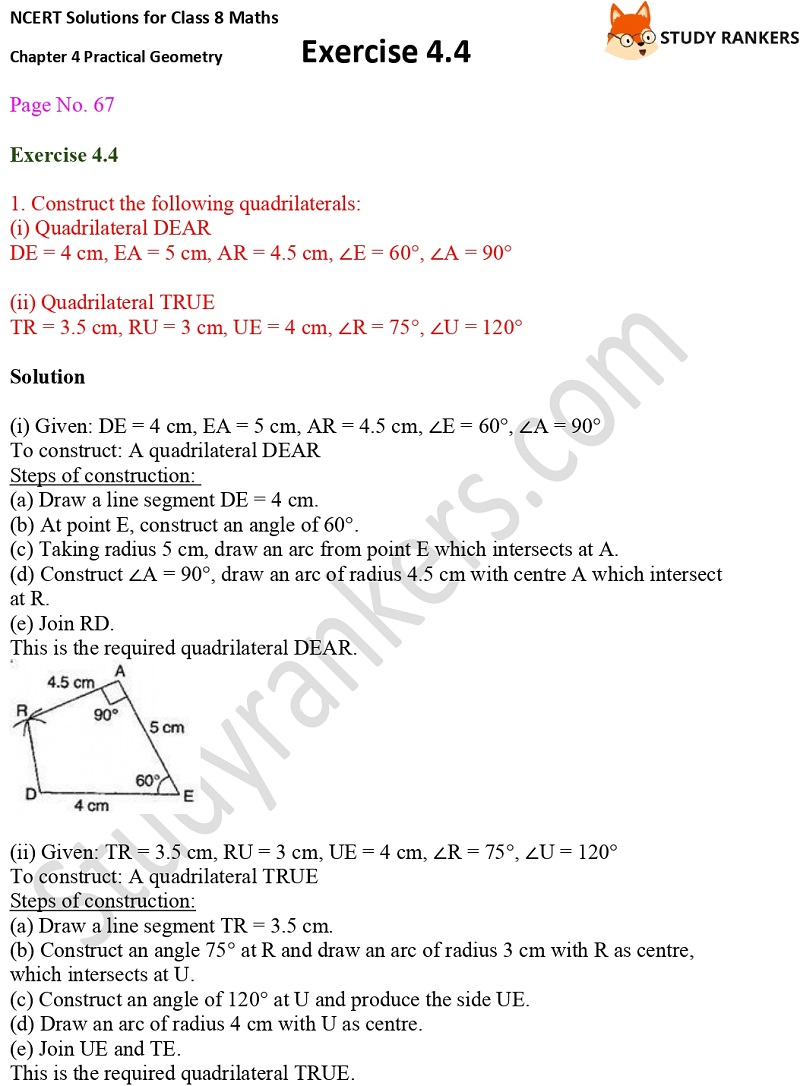>

# NCERT Solutions for Class 8 Maths Ch 4 Practical Geometry Exercise 4.4

Chapter 4 Practical Geometry Exercise 4.4 Class 8 Maths NCERT Solutions is useful guide to solve difficult questions in an easy manner. If you get stuck in a questions then you can take help from the NCERT Solutions for Class 8 Maths. These NCERT Solutions will improve the learning behaviour of the students.

In the exercise 4.4, you need to construct the quadrilaterals with the given side's measures.X